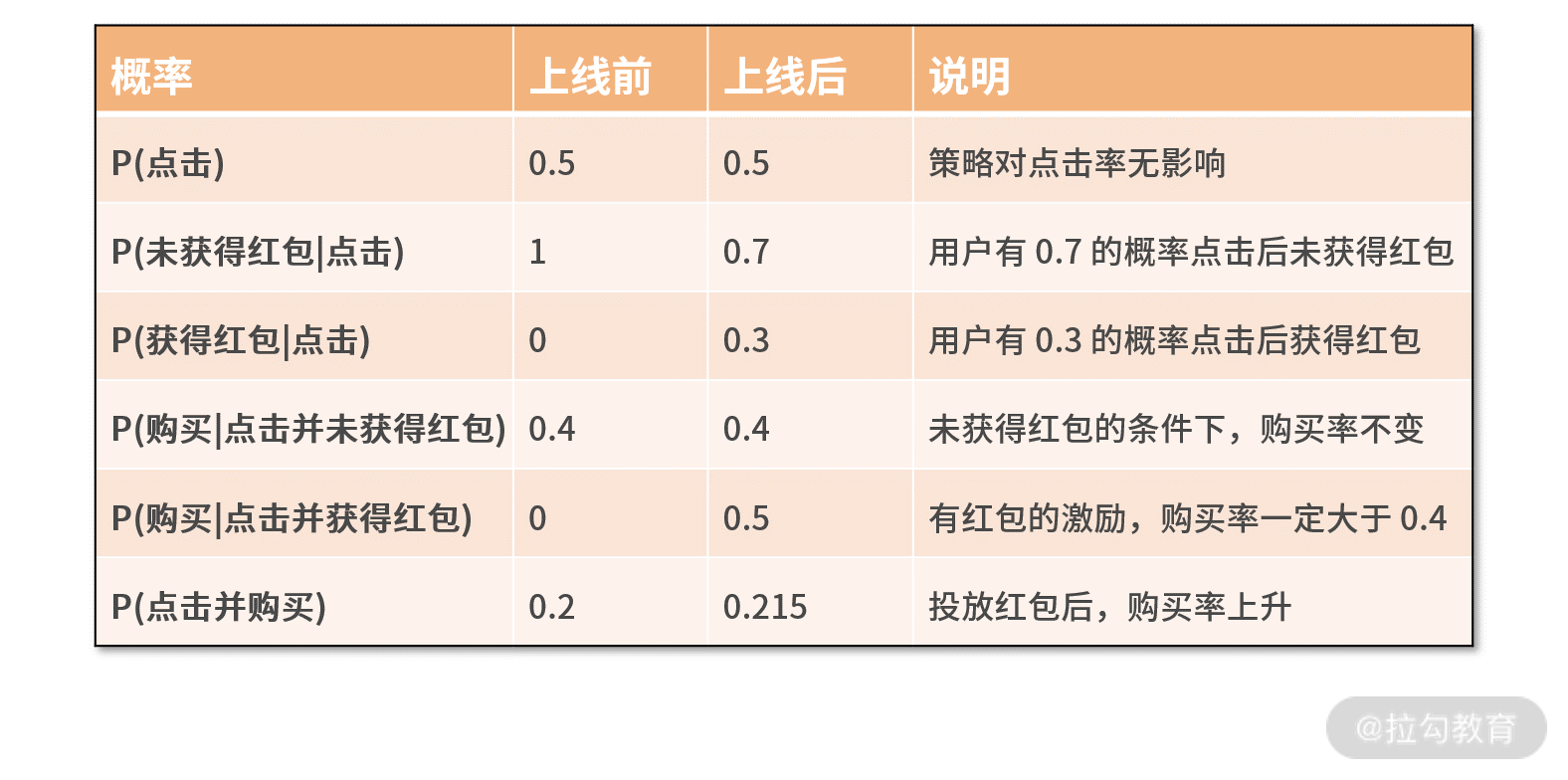08 加乘法则：如何计算复杂事件发生的概率？

概率来自统计

import random

def main():

m = 0

n = 1000

for _ in range(n):

x = random.random()

y = random.random()

if x*x + y*y < 1:

m += 1

print 1.0*m/n

if __name__ == '__main__':

main()

• 第 4、5 行定义了 m 和 n 两个变量。其中，n 赋值为 1000，意味着我们要重复执行这个动作 1000 次，m 表示坐标点落入圆内的次数；
• 接下来，就是第 6～10 行的 1000 次实验的循环了。每次实验，我们随机生成一个坐标点 (x,y)，其中 x 和 y 的取值范围都是 0～1 的浮点数；
• 这样，在第 9 行中，如果点 (x,y) 与原点的距离小于 1，则表示该点在圆内，m 自动加 1；
• 最后，打印出 m 和 n 的比值。

用加乘法则来计算复杂事件的概率

2.乘法原理

import random

obj = 0.0

for _ in range(10000):

you = random.randint(1,6)

damihu = random.randint(1,6)

if damihu == 4 and you > damihu:

obj += 1

print obj/10000

• 第 3 行的 obj，就是最终事件发生的频次；
• 我们对现象观察 10000 次，这样就形成了第 4～8 行的 for 循环；
• 每次循环，在第 5 和 6 行，随机生成你的点数和大迷糊的点数；
• 第 7 行进行判断，大迷糊为 4 点且你的点数大于大迷糊的点数；
• 如果满足条件，则在第 8 行执行 obj 加 1；
• 最终，打印出 obj 除以 10000。

条件概率

• 事件 AB 的含义是这对夫妻有女儿，且两个孩子性别相同。也就是说，这对夫妻的孩子都是女儿，即第一胎是女儿，第二胎还是女儿。此时根据乘法原理，得到 P(AB) = (1/2)×(1/2) = 1/4。
• 事件 B 为这对夫妻有女儿，不管第几胎，甚至是两胎都是女儿。这样就有了 3 种可能的情况：分别是第一胎女儿、第二胎儿子；第一胎儿子、第二胎女儿；第一胎女儿、第二胎女儿。这样根据加法原理和乘法原理，得到 P(B) = (1/2)×(1/2)+(1/2)×(1/2)+(1/2)×(1/2) = 3/4。因此 P(A|B) = P(AB) / P(B) = (1/4) / (3/4) = 1/3。

import random

fenzi = 0

fenmu = 0

for _ in range(1000):

#0 is girl; 1 is boy

first = random.randint(0,1)

second = random.randint(0,1)

if first == 1 and second == 1:

continue

else:

fenmu += 1

if first == second:

fenzi += 1

print 1.0*fenzi/fenmu

• 第 6 行开始，重复循环 1000 次。
• 第 8～9 行，随机生成两个孩子的性别。用 0 代表女儿，用 1 代表儿子。如果两个孩子都是儿子，则进行下一轮迭代。因为，这并不满足至少有一个女儿的假设条件。
• 第 12 行开始，如果有女儿，则分母加 1，如果两个孩子的性别一致，则分子也加 1。
• 最终打印出分子和分母的比值。

一个概率计算的案例

P(点击并购买) = P(点击并使用红包购买) + P(点击并未使用红包购买)

P(点击并购买) = P(购买|点击并获得红包) × P(获得红包|点击) × P(点击) + P(购买|点击并未获得红包) × P(未获得红包|点击) × P(点击)• 投放红包是在点击之后，因此对点击率无影响；
• 用户点击商品详情页的条件下，获得红包的概率是 0.3，未获得红包的概率是 0.7；
• 对于未获得红包的用户，其购买率与实验前一致，都是 0.4。对于获得红包的用户，其购买率会上升，达到 0.5。

小结

• 概率来自统计。当你束手无策时，不妨从多次的重复试验中，统计目标事件出现的频次，来估算概率。
• 加乘法则是计算概率的有力手腕。对复杂事件按照并行或串行来拆解，再利用加乘法则就可以完成复杂事件的概率计算。
• 条件概率是处理有关联事件的方法。虽然条件概率有些晦涩，但牢牢记住定义式 P(A|B) = P(AB) / P(B)，就能让条件概率转换为普通事件的概率。在实际应用中，一定要耐着性子，仔细琢磨事件背后的相关关系，再利用这些方法，就能把概率计算清楚。